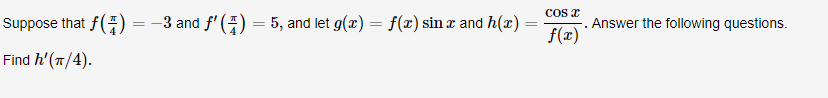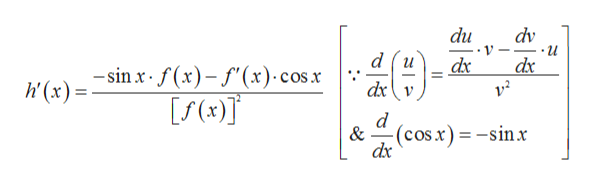# COSSuppose that f(=-3 and f(- 5, and let g(x)f(x) sinx and h(x) =Answer the following questionsFind h'(T/4)

Question

Find h'(π/4).help_outlineImage TranscriptioncloseCOS Suppose that f( =-3 and f( - 5, and let g(x) f(x) sinx and h(x) = Answer the following questions Find h'(T/4) fullscreen
check_circle

Step 1

Given,

Step 2

Differentiating h(x) with r...help_outlineImage Transcriptionclosedu dv d (и dxv dx dx -sinx f(x)-f(x) cosx h'(x) d & -(cos.x)-sinx dx fullscreen

### Want to see the full answer?

See Solution

#### Want to see this answer and more?

Solutions are written by subject experts who are available 24/7. Questions are typically answered within 1 hour.*

See Solution
*Response times may vary by subject and question.
Tagged in

### Derivative